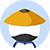If ABCD is a rectangle, BC = x and AB = 2x, then the circumference of the circle, in terms of x, is• A$\sqrt{3}$x$\pi$
• B$\sqrt{5}$x$\pi$
• C$\sqrt{3x}$$\pi$
• D$\sqrt{5x}$$\pi$
• E$5x^{2}\pi$

• KMF解析
• 网友解析

### 题目讨论

• 优质讨论
• 最新讨论